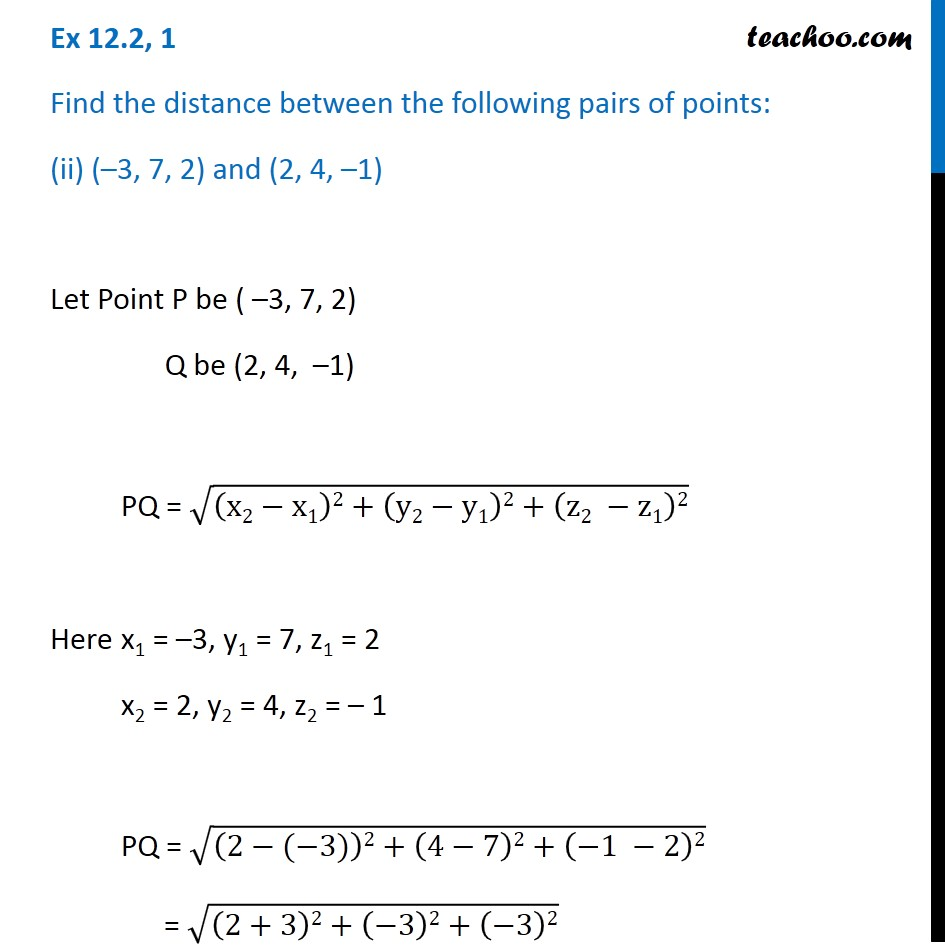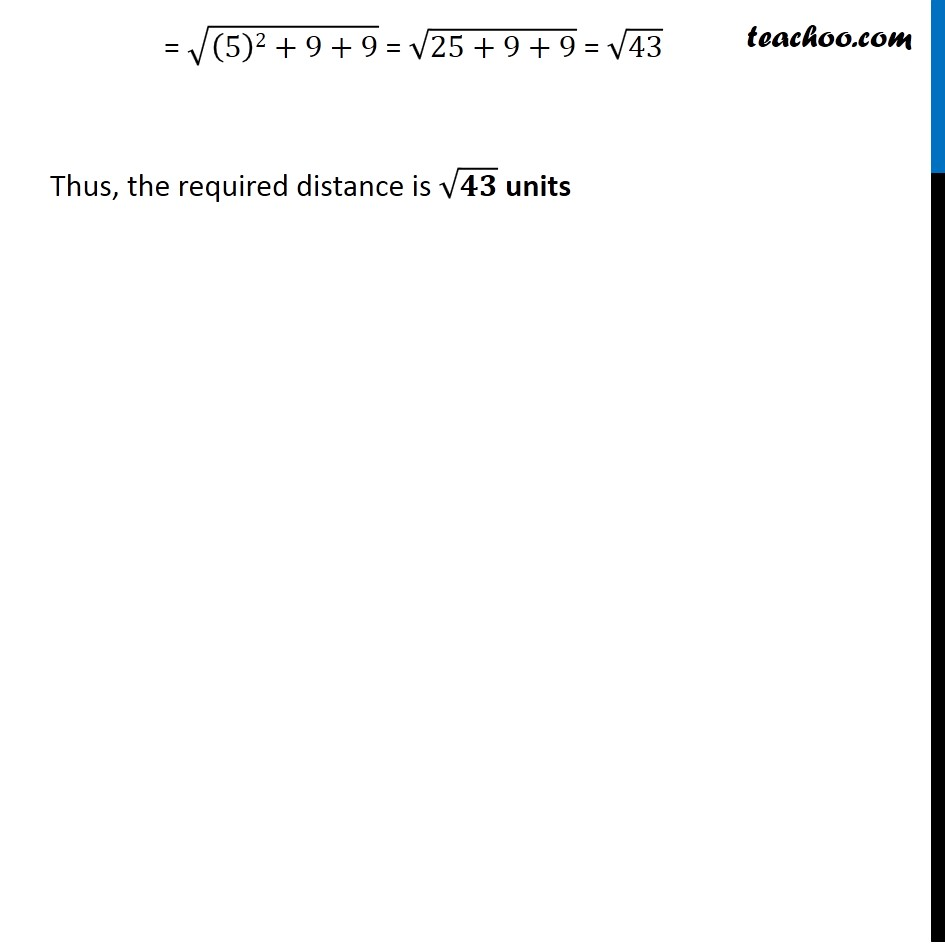Ex 11.2

Chapter 11 Class 11 - Intro to Three Dimensional Geometry
Serial order wiseLearn in your speed, with individual attention - Teachoo Maths 1-on-1 Class

### Transcript

Ex 11.2, 1 Find the distance between the following pairs of points: (ii) (–3, 7, 2) and (2, 4, –1) Let Point P be ( –3, 7, 2) Q be (2, 4, –1) PQ = √((x2−x1)2+(y2−y1)2+(z2 −z1)2) Here x1 = –3, y1 = 7, z1 = 2 x2 = 2, y2 = 4, z2 = – 1 PQ = √((2−(−3))2+(4−7)2+(−1 −2)2) = √((2+3)2+(−3)2+(−3)2) = √((5)2+9+9) = √(25+9+9) = √43 Thus, the required distance is √𝟒𝟑 units